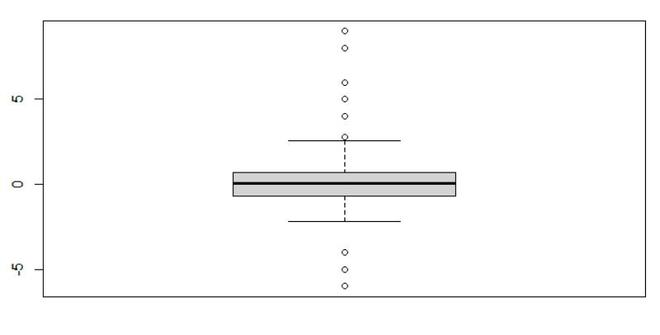GeeksforGeeks App
Open AppBrowser
Continue

# Remove Outliers from Data Set in R

In this article, we will be looking at the approach to remove the Outliers from the data set using the in-built functions in the R programming language.

Outliers are data points that don’t fit the pattern of the rest of the data set. The best way to detect the outliers in the given data set is to plot the boxplot of the data set and the point located outside the box in the boxplot are all the outliers in the data set. In this approach to remove the outliers from the given data set, the user needs to just plot the boxplot of the given data set using the simple boxplot() function, and if found the presence of the outliers in the given data the user needs to call the boxplot.stats() function which is a base function of the R language, and pass the required parameters into this function, which will further lead to the removal of the outliers present in the given data sets.

boxplot.stats() function is typically called by another function to gather the statistics necessary for producing box plots but may be invoked separately.

Syntax: boxplot.stats(x, coef = 1.5, do.conf = TRUE, do.out = TRUE)

Parameters:

• x: a numeric vector for which the boxplot will be constructed.
• coef: determines how far the plot ‘whiskers’ extend out from the box.
• do.conf, do.out: logicals; if FALSE, the conf or out component respectively will be empty in the result.

Let us first look at a regular plot without removing the outliers.

Example: Initial plot

## R

 `gfg<-``rnorm``(500)`` ` `gfg[1:10]<-``c``(-4,2,5,6,4,1,-5,8,9,-6)`` ` `boxplot``(gfg)`

Output:Now let us again visualize the above plot but this time without outliers by applying the given approach.

Example: Removing Outliers Using boxplot.stats() Function-

## R

 `gfg<-``rnorm``(500)`` ` `gfg[1:10]<-``c``(-4,2,5,6,4,1,-5,8,9,-6)`` ` `gfg <- gfg[!gfg %``in``% ``boxplot.stats``(gfg)\$out]   `` ` `boxplot``(gfg)`

Output:My Personal Notes arrow_drop_up